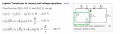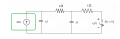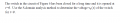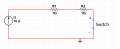# Conversion of voltage or current sources to Laplace transform equivalent

#### ptzmm

Joined Oct 6, 2014
2
Hello all,
I am really having a hard time understanding Laplace transform conversion of independent voltage or current sources. I understand that for voltage or current connected in series with the switch, we can assume this as step function and after conversion to Laplace transform, result will be V/s or I/s according to wiki (the first picture).
However, in this particular homework, there is no switch being connected to 10A current source. I don't know whether Laplace transform equivalent is simply 10A or (10/s)A.
Would you mind helping me to clear my doubt?
Thank you.#### t_n_k

Joined Mar 6, 2009
5,455
In the case of the 10A source in the lower circuit the 10A source has two roles.
Before time t = 0 seconds it sets the initial conditions in the circuit. One assumes it has been supplying current for an infinite time prior to the switch 'S' being opened at t=0 seconds.
After time t = 0 seconds when the switch 'S' opens, it contributes to the transient response.
So it will still be assigned as 10/s A in the Laplace domain for the purpose of finding the transient response from t=0 onward.
In the situation where the source is intended to be connected to a circuit at t=0, then one would place the source in parallel with a switch which opens at t=0, when it is explicitly intended to show the current is supplied only at that time.
Alternatively, the current might be denoted as 10u(t) A, where the function u(t) is the Heaviside unit step function and which formally denotes that the 10A current "exists" only for t>=0 seconds.

•ptzmm

#### ptzmm

Joined Oct 6, 2014
2This is the question asking voltage across switch at t>0.
When considering for initial conditions, should equivalent circuit look like this as capacitors are open circuit under DC conditions?Since resistance of the switch is unknown, no matter what the current will be (in this case 10A), the initial condition of Vs will be 0.
Is my assumption correct?
Thank you.

#### t_n_k

Joined Mar 6, 2009
5,455
No that is definitely not the correct equivalent circuit for initial conditions.
The value initially seen across the switch when opened will be the voltage across the right hand capacitor. What do you think this capacitor voltage will be, immediately before the switch is opened? Hence immediately after? Remember a capacitor voltage cannot change instantaneously.

#### Jun Wei

Joined Oct 6, 2014
1

#### MrAl

Joined Jun 17, 2014
10,590
Hi,

As tnk is pointing out, first you have to solve for the initial conditions across each capacitor before the switch is opened. So you basically have two circuits to solve: one where the switch is closed (can caps have infinite time to charge up to their appropriate voltages) and one where the switch is just opened (which will require knowing the two cap voltages from the previous circuit).

#### WBahn

Joined Mar 31, 2012
29,149
The key to getting this straight is to remember that you are working with the single-sided Laplace transform, which means that the circuit component you are transforming is, by definition, forced to be zero for all time less than zero. When you have a circuit element that is not zero for t<0 AND that fact influences the behavior of the circuit for t >= 0, you have to add in additional elements that serve to impose the initial conditions at t=0.